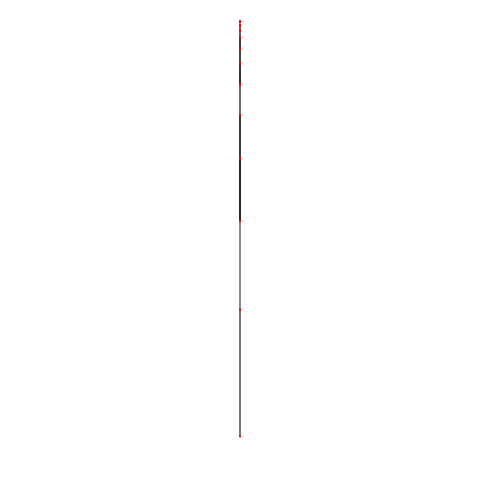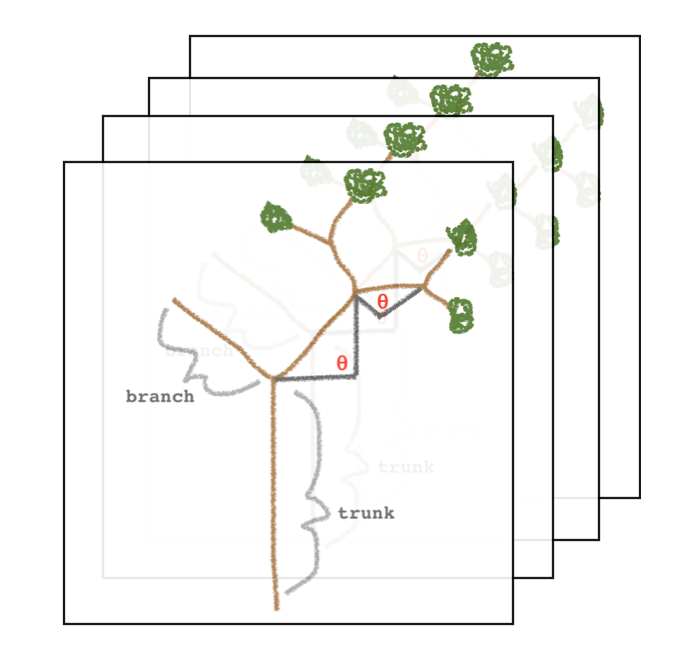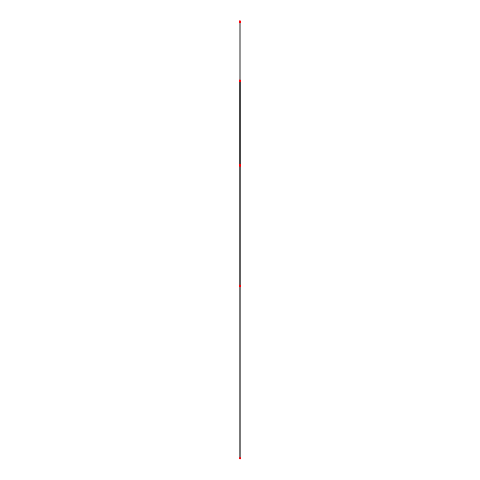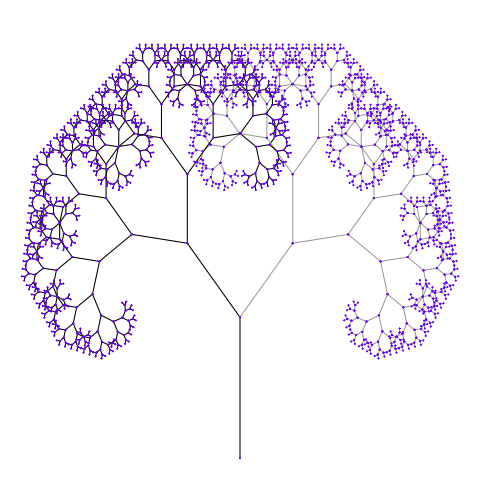Programmer/Data Scientist/Instructor・Mostly write Python & R・Big fan of OpenCV & p5js

Home

# OOP Fractal Trees in R with R6, ggplot2, & gganimate (part 2)

Published Oct 08, 2018

### Share

This post is part 2 of 2, and, as stated in part 1, the end goal is to create an animated fractal tree with `R6` & `gganimate`. Today, we will be animating the `fractal_tree` `R6` class from part 1 with `gganimate`.

The below code and plot show where we’re going to get by the end of this post.

``````# Create & animate R6 tree object
tree = fractal_tree_seq\$new()
tree\$animate()
``````Note: This post is meant to explore `R6` functionality; it’s not claiming to be the best way to create our fractal trees. Some design choices were solely to leverage varied features. Additionally, this post is more example-based than explanation based. For more in-depth explanations, I recomend going to this page from `R6` or check out this chapter from Advanced R

# DesignWe already have a `fractal_tree` object that can create a single tree with branches positioned at a given angle. For simplicity, we will build our animated tree as a sequence of our (already defined) `fractal_tree` objects; each tree will be a frame in the animation. Disclaimer: if you end up running this code, you’ll see that this approach might not be the most efficient approach, but it works.

# Implementation

Note: This post’s code assumes that the objects from part 1 are loaded into your R session. The complete code from part 1 can be found here

Sticking with the theme of the 2 part series, we’ll create a single `R6` class to house our animation process. The “Design” section above might seem to be written at a very high level, but it covers almost all of the implementation details that we’ll discuss below.

### fractal_tree_seq

The object doing the animation is given the name `fractal_tree_seq`, since it is simply a sequence of `fractal_tree`s. In the `initialize` method of the object, we loop the the user provided `angle_seq` and create a tree at each angle in the sequence. Additionally, when we create each tree, we assign some meta data that shows which frame the tree belongs to. Lastly, in our `initialize` method we assign a color to each angle, this info will be used in plotting to give our animation some flare.

To wrap up our `fractal_tree_seq` class we add a `public` `animate` method that looks a lot like the plot method from part 1. The syntax of `gganimate` is very similiar to `ggplot2`’s, so experience with the later should make the `animate` code feel familiar. The only bit of `gganimate` code we add to the `ggplot2` expression is `+ transition_manual(frame)`. This command will use the frame data we assigned in `initialize` to create a gif of our `fractal_tree`s.

And that’s it! We acheived the goal of to creating and animating a fractal tree with `R6` and `gganimate`.

``````fractal_tree_seq = R6Class('fractal_tree_seq',
public = list(
trees = data.frame(),
initialize = function(trunk_len = 10,
angle_seq = seq(0, 2 * pi - pi / 32, pi / 32),
len_decay = 0.7,
min_len = 0.25,
verbose = TRUE) {
total = length(angle_seq)
for (i in seq_along(angle_seq)) {
if (verbose) cat(sprintf('creating tree %s of %s\n', i, total))
angle = angle_seq[i]

tree_i = fractal_tree\$new(trunk_len = trunk_len,
delta_angle = angle,
len_decay = len_decay,
min_len = min_len)

branches_i = tree_i\$branches
branches_i\$angle = angle
branches_i\$frame = i

self\$trees = rbind(self\$trees, branches_i)
}

angle_colors = data.frame(angle = sort(unique(self\$trees\$angle)))
angle_colors\$angle_color = rainbow(nrow(angle_colors))

self\$trees = merge(self\$trees, angle_colors, all.x = TRUE, by = 'angle')
},  # initialize

animate = function() {
ggplot(self\$trees, aes(x, y, group = id)) +
geom_line(aes(color = branch_color)) +
geom_point(size = .2, aes(color = angle_color)) +
scale_color_identity() +
guides(color = FALSE, linetype = FALSE) +
theme_void() +
transition_manual(frame)
}
)  # public
)  #fractal_tree_seq
``````

# Final Product

This last section will be a few examples of using the functionality of our `fractal_tree` class.

``````# Create & animate R6 tree object
tree = fractal_tree_seq\$new()
tree\$animate()
``````
``````# Create & animate R6 tree object with new min_len
tree = fractal_tree_seq\$new(min_len = 3)
tree\$animate()
````````````# Create & animate R6 tree object with new angle_seq
tree_seq = fractal_tree_seq\$new(angle_seq = runif(4, min=pi / 16, max=pi / 4))
tree\$animate()
``````

*made smaller since it’s so distracting